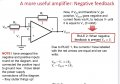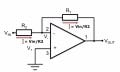# Op Amps Use?

#### JackBidds

Joined Jan 10, 2021
17
Hi everyone, we’ve just started looking at Op Amps in my University Physics Course and in particular negative feedback. i have been given a rule that in the circuit below because V+ is connected to ground then V- and V+ will both be equal to 0v. If this is the case then what is the point in the circuit, it seems to me like the current just flows through the resistor at the top and no voltage gets amplified. If someone could explain this to me I would be very grateful.
Thanks Everyone#### dl324

Joined Mar 30, 2015
15,838
If this is the case then what is the point in the circuit, it seems to me like the current just flows through the resistor at the top and no voltage gets amplified. If someone could explain this to me I would be very grateful.
You're correct in saying that the current just flows through the two resistors in an ideal opamp. Do the arithmetic to determine what the output voltage would be.

If R1 = R2, what is the output voltage?

#### JackBidds

Joined Jan 10, 2021
17
You're correct in saying that the current just flows through the two resistors in an ideal opamp. Do the arithmetic to determine what the output voltage would be.

If R1 = R2, what is the output voltage?
Vin - I(R1 + R2) ?

#### dl324

Joined Mar 30, 2015
15,838
Vin - I(R1 + R2) ?
If R1 = R2, can you express Vout only as a function of Vin?

#### JackBidds

Joined Jan 10, 2021
17
If R1 = R2, can you express Vout only as a function of Vin?
I’m not sure

#### dl324

Joined Mar 30, 2015
15,838
I’m not sure
Since the inverting input of the opamp is at virtual ground, the voltage drop across R1 is Vin. If R2 = R1, what is the voltage drop across R2?

#### JackBidds

Joined Jan 10, 2021
17
Why is the voltage drop at R1 Vin? Wouldn’t it be a share of Vin/

#### dl324

Joined Mar 30, 2015
15,838
Why is the voltage drop at R1 Vin? Wouldn’t it be a share of Vin/
R1 is the only resistor across Vin. Why do you think it would be divided with R2?

#### Papabravo

Joined Feb 24, 2006
20,394
The op amp, looks at the voltage difference between it's inputs. Without feedback, in the open loop configuration, consider what happens when the gain is on the order of 100,000. If V+ is at GROUND and V- is at -100 μV, then [0 - (-100 μV)] x 100,000 = 10 Volts. The output of the opamp will always be limited by the power supply rails. Similarly if V- is at +100 μV you would have [0 - 100 μV] x 100,000 = -10 volts.

In the case of feedback, the opamp will look at the difference in the inputs, and set the output voltage in such a way as to make the differential voltage across the inputs as close to zero as it possibly can. The phrase "as close to zero as possible" is why the differential inpust to an op amp are sometimes referred to as a "virtual ground". It is not an actual ground but pretty darn close to a 1st order approximation.

It has many potential uses, be patient and understanding will come.

You are correct in concluding that all the current flows in the resistors, The input currents to an op amp are tiny: sometimes as small as a few picoamps, maybe smaller in some cases.

Last edited:

#### JackBidds

Joined Jan 10, 2021
17
R1 is the only resistor across Vin. Why do you think it would be divided with R2?
I thought because no current goes to V- the voltage would be split between R1 and R2

#### Papabravo

Joined Feb 24, 2006
20,394
Why is the voltage drop at R1 Vin? Wouldn’t it be a share of Vin/
Because the other side of R1, on the V- terminal input to the op amp, is at the potential of the virtual ground created by the op amp output.

KCL says the sum of the currents into and out of a node must be zero. There is current through R1 and current through R2, and nothing into the op amp input. Ergo I(R1) = -I(R2). Apply Ohms law to get"
$\frac{V_{in}}{R1}\;=\;-\frac{V_{out}}{R2}$
The negative sign is there because the op amp's output is inverted. Clearly if the resistors are equal the voltages will equal, but with the opposite sign. Also you can see that if R2 is bigger than R1 there is gain, and if it is smaller there is attenuation.

This enormous fact was recognized in the 1930's, that you could build an amplifier whose gain does not depend on the characteristics of the active device (a vacuum tube, transistor, or other semiconductor device) but only on the passive components whose values are much easier to control reliably and repeatedly. The history of this discovery but reserrchers at Bell Labs and other places is fascinating.

Last edited:

#### dl324

Joined Mar 30, 2015
15,838
I thought because no current goes to V- the voltage would be split between R1 and R2
But we've established that the inverting input is at virtual ground.

#### ApacheKid

Joined Jan 12, 2015
1,269
I'm following this (and won't butt in, but I'm also a novice with these, only read about them).

I want to do some experiments with them, breadboard and stuff to reinforce my understanding.

#### JackBidds

Joined Jan 10, 2021
17
But we've established that the inverting input is at virtual ground.
Yes?

#### ApacheKid

Joined Jan 12, 2015
1,269
Does this simple setup introduce any phase change? I guess not - but is that right?

#### dl324

Joined Mar 30, 2015
15,838
If the drop across R1 is Vin and R2 = R1, what is the output voltage?

#### JackBidds

Joined Jan 10, 2021
17
If the drop across R1 is Vin and R2 = R1, what is the output voltage?
Man I don’t understand, if no current flows through V- then why isn’t the current just treated as a line going from Vin thro8gh both resistors to Vout? Thus R1 and R2 would both get an equal share 1/2 Vin

#### dl324

Joined Mar 30, 2015
15,838
Man I don’t understand, if no current flows through V- then why isn’t the current just treated as a line going from Vin thro8gh both resistors to Vout? Thus R1 and R2 would both get an equal share 1/2 Vin
I reversed the resistors. Normally we'd number them from left to right, but your schematic doesn't do that. I meant that Vin is across R2.What is the voltage across R1?

#### Papabravo

Joined Feb 24, 2006
20,394
Does this simple setup introduce any phase change? I guess not - but is that right?
At low frequencies the answer is no. At higher frequencies, the addition of capacitors and the AC characteristics of the amplifier make the answer more complicated. Simulators like LTspice are a great way to learn with a minimum of aggravation. There is no substitute for the breadboard when you actually go to do something.

Last edited:

#### MrChips

Joined Oct 2, 2009
29,241
I'm following this (and won't butt in, but I'm also a novice with these, only read about them).

I want to do some experiments with them, breadboard and stuff to reinforce my understanding.